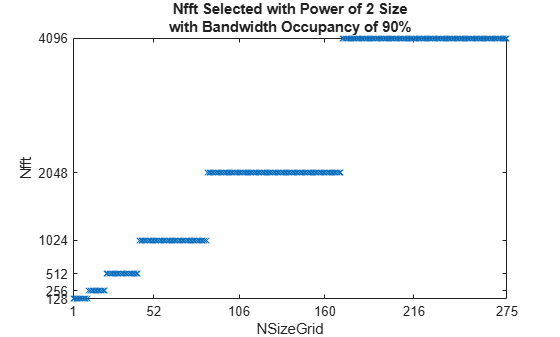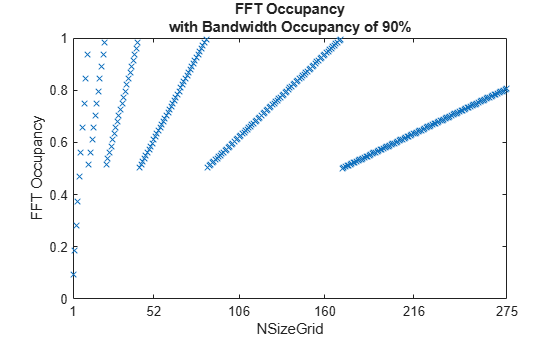Use Custom OFDM Sample Rate and Custom FFT Size

This example explains how to set custom values for the sample rate input, SampleRate, and the fast Fourier transform (FFT) size input, Nfft, when you call an OFDM function (nrOFDMModulate, nrOFDMInfo, and nrOFDMDemodulate).

Custom OFDM Sample Rate

The value that you set for the SampleRate input determines the sample rate of the waveform.

The nominal sample rate corresponding to the FFT size used in the OFDM modulation, ${\mathit{FFT}}_{\mathit{SR}}$, is equal to Nfft * carrier.SubcarrierSpacing * 1000, where carrier is the input argument of the function call, specifying the carrier configuration.

Because the resampling of the OFDM-modulated waveform is by a factor of SampleRate/${\mathit{FFT}}_{\mathit{SR}}$, the resampling is costly if SampleRate and ${\mathit{FFT}}_{\mathit{SR}}$ do not have large common factors.

Custom FFT Size

The value that you set for the Nfft input must satisfy these conditions.

• Nfft is an integer value resulting in integer-valued cyclic prefix lengths.

• Nfft is an integer power of 2.

• Nfft results in a maximum occupancy of 100%. The actual occupancy is equal to carrier.NSizeGrid * 12 / Nfft.

You can only achieve a bandwidth occupancy of exactly 100% when these conditions apply.

• The FFT and the carrier grid have the same size, that is, Nfft = carrier.NSizeGrid * 12.

• Resampling is not needed, that is, SampleRate = Nfft * carrier.SubcarrierSpacing * 1000.

Plot Bandwidth Occupancy

Create a carrier configuration object.

carrier = nrCarrierConfig;

Set the SampleRate for each NSizeGrid such that the bandwidth occupancy (txBW / SampleRate) is 90%. Set Nfft to a power of 2.

nSizeGrids = 1:275;
userSampleRateUserNfft = zeros(1,275);
fftOccupancy = zeros(1,275);
for nSizeGrid = 1:275
carrier.NSizeGrid = nSizeGrid;
% Transmission bandwidth of OFDM waveform
txBW = carrier.NSizeGrid * 12 * carrier.SubcarrierSpacing * 1000;
sr = txBW / 0.9;
nfft = max(128,2^ceil(log2(carrier.NSizeGrid * 12)));
ofdmInfo = nrOFDMInfo(carrier,'SampleRate',sr,'Nfft',nfft);
userSampleRateUserNfft(nSizeGrid) = ofdmInfo.Nfft;
fftOccupancy(nSizeGrid) = carrier.NSizeGrid * 12 / ofdmInfo.Nfft;
end

Plot the resulting FFT size.

figure;
plot(nSizeGrids,userSampleRateUserNfft,'x');
title({'Nfft Selected with Power of 2 Size' 'with Bandwidth Occupancy of 90%'});
axis([1 275 min(userSampleRateUserNfft) max(userSampleRateUserNfft)]);
xlabel('NSizeGrid');
xticks([1 52 106 160 216 275]);
ylabel('Nfft');
yticks(2.^(7:12));Plot the resulting FFT occupancy.

figure;
plot(nSizeGrids,fftOccupancy,'x');
title({'FFT Occupancy' 'with Bandwidth Occupancy of 90%'});
axis([1 275 0 1]);
xlabel('NSizeGrid');
xticks([1 52 106 160 216 275]);
ylabel('FFT Occupancy');Get OFDM Information

Update the carrier to 25 resource blocks (RBs).

carrier.NSizeGrid = 25;

Get OFDM information.

ofdmInfo = nrOFDMInfo(carrier)
ofdmInfo = struct with fields:
Nfft: 512
SampleRate: 7680000
CyclicPrefixLengths: [40 36 36 36 36 36 36 40 36 36 36 36 36 36]
SymbolLengths: [552 548 548 548 548 548 548 552 548 548 548 ... ]
Windowing: 18
SymbolPhases: [0 0 0 0 0 0 0 0 0 0 0 0 0 0]
SymbolsPerSlot: 14
SlotsPerSubframe: 1
SlotsPerFrame: 10

The CyclicPrefixLengths and SymbolLengths fields in the output structure return the cyclic prefix lengths and total OFDM symbol lengths for each OFDM symbol in a subframe. The total OFDM symbol length is composed of the cyclic prefix and the nominal OFDM symbol length equal to the FFT size.

ofdmInfo.SymbolLengths - ofdmInfo.CyclicPrefixLengths
ans = 1×14

512   512   512   512   512   512   512   512   512   512   512   512   512   512

Because OFDM symbol construction is performed using an IFFT of size that you specify in the Nfft input, the CyclicPrefixLengths and SymbolLengths fields return values in terms of this Nfft value. The specified Nfft is also returned in the Nfft field of the output.

nfft = 640;
ofdmInfo = nrOFDMInfo(carrier,'Nfft',nfft)
ofdmInfo = struct with fields:
Nfft: 640
SampleRate: 9600000
CyclicPrefixLengths: [50 45 45 45 45 45 45 50 45 45 45 45 45 45]
SymbolLengths: [690 685 685 685 685 685 685 690 685 685 685 ... ]
Windowing: 22
SymbolPhases: [0 0 0 0 0 0 0 0 0 0 0 0 0 0]
SymbolsPerSlot: 14
SlotsPerSubframe: 1
SlotsPerFrame: 10

ofdmInfo.SymbolLengths - ofdmInfo.CyclicPrefixLengths
ans = 1×14

640   640   640   640   640   640   640   640   640   640   640   640   640   640

For the default sample rate, the number of time-domain samples of each OFDM symbol matches the values of SymbolLengths field. If you specify the SampleRate input, the specified value is returned in the SampleRate field of the output. However, the CyclicPrefixLengths and SymbolLengths fields are expressed in terms of the IFFT size used during OFDM symbol construction, therefore, these values do not change in the output. The waveform is resampled to the sample rate that you specify after OFDM symbol construction. Depending on the sample rate, the length of the cyclic prefixes and nominal OFDM symbols in the corresponding OFDM waveform may not be an integer number of samples.

sr = 1.35 * nfft * carrier.SubcarrierSpacing * 1e3;
ofdmInfo = nrOFDMInfo(carrier,'Nfft',640,'SampleRate',sr)
ofdmInfo = struct with fields:
Nfft: 640
SampleRate: 12960000
CyclicPrefixLengths: [50 45 45 45 45 45 45 50 45 45 45 45 45 45]
SymbolLengths: [690 685 685 685 685 685 685 690 685 685 685 ... ]
Windowing: 22
SymbolPhases: [0 0 0 0 0 0 0 0 0 0 0 0 0 0]
SymbolsPerSlot: 14
SlotsPerSubframe: 1
SlotsPerFrame: 10

ratio = ofdmInfo.SampleRate / (ofdmInfo.Nfft * carrier.SubcarrierSpacing * 1e3)
ratio = 1.3500
disp(num2str(ofdmInfo.SymbolLengths*ratio,'%0.3f '));
931.500 924.750 924.750 924.750 924.750 924.750 924.750 931.500 924.750 924.750 924.750 924.750 924.750 924.750